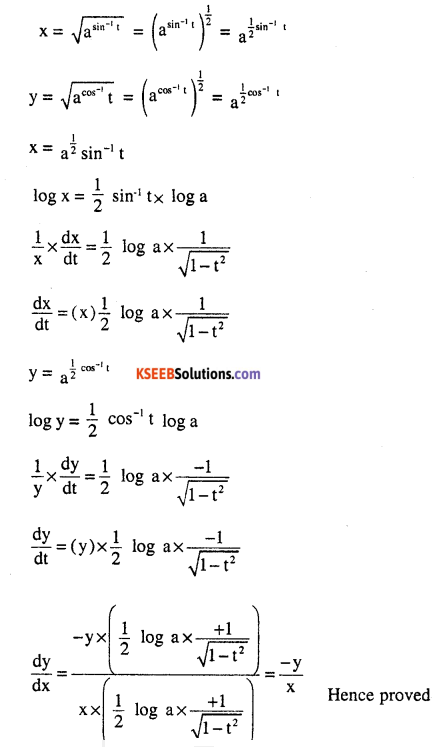# 2nd PUC Maths Question Bank Chapter 5 Continuity and Differentiability Ex 5.6

Students can Download Maths Chapter 5 Continuity and Differentiability Ex 5.3 Questions and Answers, Notes Pdf, 2nd PUC Maths Question Bank with Answers helps you to revise the complete Karnataka State Board Syllabus and score more marks in your examinations.

## Karnataka 2nd PUC Maths Question Bank Chapter 5 Continuity and Differentiability Ex 5.6

### 2nd PUC Maths Continuity and Differentiability NCERT Text Book Questions and Answers Ex 5.6

If x and y are connected parametrically by the equations given in Exercises 1 to 10, without eliminating the parameter,find $$\frac{\mathrm{d} y}{\mathrm{d} x}$$

Question 1.
x = 2at2, y = at4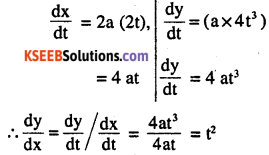Question 2.
x = a cos θ, y = b cos θ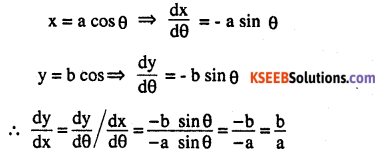Question 3.
x = sin t , y = cos 2t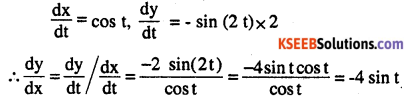Question 4.
$$x=4 t, y=\frac{4}{t}$$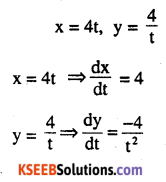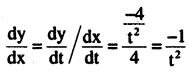Question 5.
x = cosθ – cos 2θ y = sinθ – sin 2θ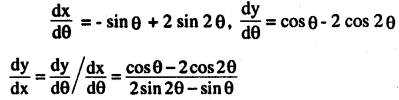Question 6.
x = a (θ – sin θ),y = (1 + cos θ)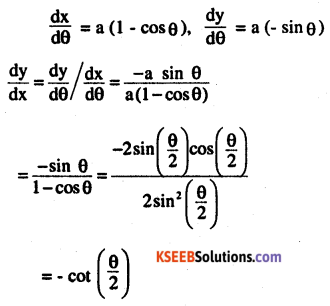Question 7.
$$x=\frac{\sin ^{3} t}{\sqrt{\cos 2 t}}, y=\frac{\cos ^{3} t}{\sqrt{\cos 2 t}}$$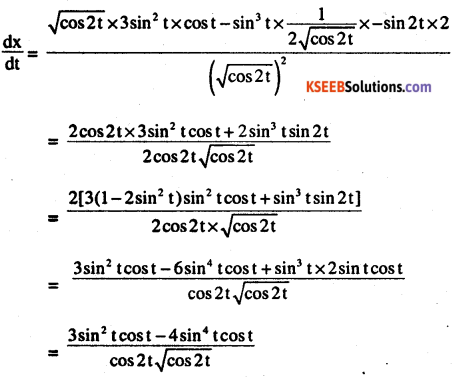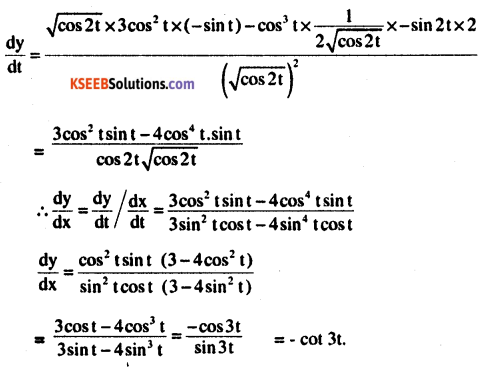Question 8.
$$x=a\left(\cos t+\log \tan \frac{t}{2}\right), y=a \sin t$$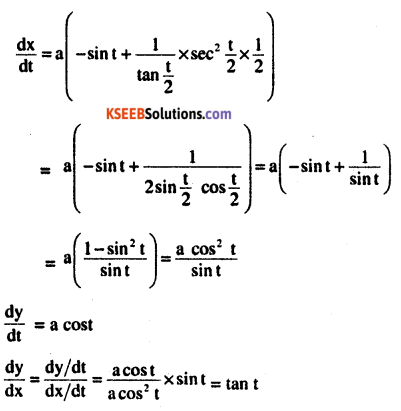Question 9.
x = a sec θ, y = b tan θ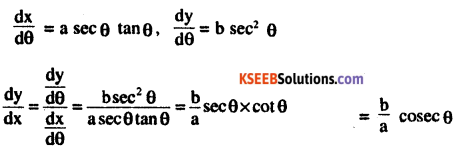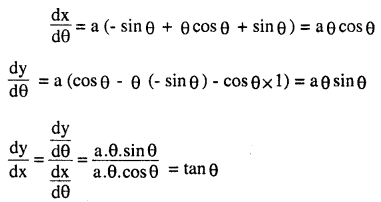$$\text { If } x=\sqrt{a^{\sin ^{-1} t}}, y=\sqrt{a^{\cos ^{-1} t}}, \text { show that } \frac{d y}{d x}=-\frac{y}{x}$$